# Decompose the E field into conservative and non-conservative parts

• B
Homework Helper
2022 Award
In the example below, we can write the instant result 0.2V without solving complex voltage loop equations.

If all you have in your toolbox is a hammer, everything begins to look like a nail.
One can likely contrive situations where this approach has hueristic appeal. In general, for reasons well stated, it is a "solution" in search of a problem. For your symmetry argument to hold, the entire magnetic universe would need to be cylindrically symmetric. Might be tough.
Stokes theorem deals with closed curves for a reason

•vanhees71
alan123hk
@vanhees71
I'm not trying to describe the non-existent potential created by the induced electric field, but the scalar field created by the accumulated charge on this circuit.

As is the case in this paper http://kirkmcd.princeton.edu/examples/lewin.pdf , the same circuits are also described with potentials. Quoted below.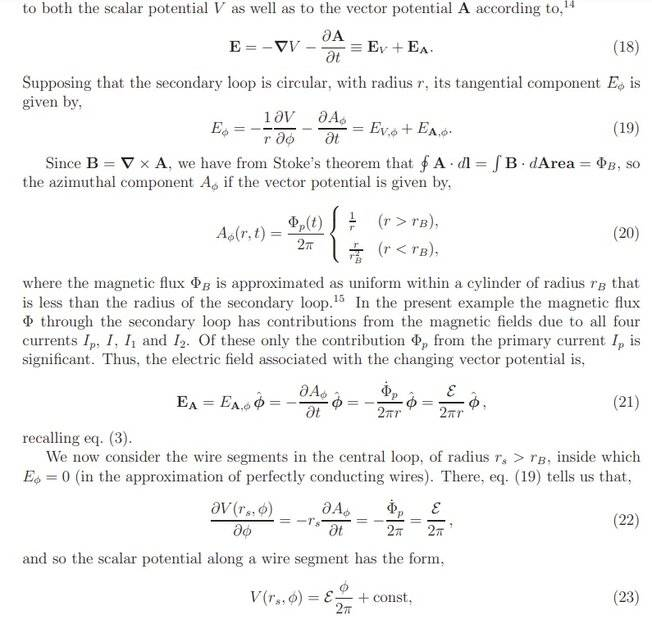alan123hk
The following example explicitly shows the presence of a scalar field generated in the circuit.
Note that the direction of the circular induced electric field is at right angles to the direction of the wires connected to the voltmeter, so the induced electric field does not generate any emf on the wires. Now look at a plane at right angles to the ring circuit. If the voltmeter and its connecting wires are moved to any position on the plane, the displayed reading will not change, because the direction of the two wires is always at right angles to the induced electric field, so it must be measuring the potential difference generated by the accumulated charge in the circuit.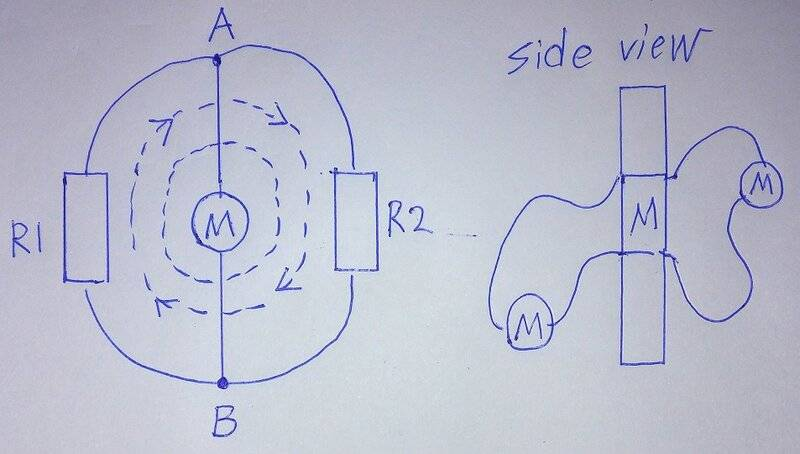Some people may say why to complicate things, it seems that it has no practical value. But I disagree, like the above example, at least we understand what is measured and why the voltage change is measured when the wire connected to the voltmeter deviates from this plane, maybe it can be used to detect whether the plane in the mechanical structure deviates from the original position.Last edited:
SDL
As is the case in this paper http://kirkmcd.princeton.edu/examples/lewin.pdf , the same circuits are also described with potentials. Quoted below.
The last equation ##V=\epsilon \frac \phi {2 \pi}+const## is obviously false, because here V for angle zero is either undefined or experiances a leap. But any scalar potential function has to be continuous.

Homework Helper
2022 Award
Some people may say why to complicate things, it seems that it has no practical value. But I disagree, like the above example, at least we understand why the voltage change is measured when the wire connected to the voltmeter deviates from this plane, maybe it can be used to detect whether the plane in the mechanical structure deviates from the original position.Two practical points:
The indiced emf is proportional to the time derivative of the flux and therefore seldom constant and happy
A standard form of magnetic field sensor (prior to hall effect sensors and FETs) was the flip coil.
Generally the fact that one can perform a Helmholtz decomposition of any well behaved vector field is not news and, while occasionally useful, it should not be extorted with the hammer.

alan123hk
The last equation V=ϵϕ2π+const is obviously false, because here V for angle zero is either undefined or experiances a leap. But any scalar potential function has to be continuous.
Sorry, I really don't understand why this is going wrong. This constant means that when the angle is 0, V can be any value. This is a boundary condition and the user may decide to substitute any value.

alan123hk
Two practical points:
The indiced emf is proportional to the time derivative of the flux and therefore seldom constant and happy
A standard form of magnetic field sensor (prior to hall effect sensors and FETs) was the flip coil.
Generally the fact that one can perform a Helmholtz decomposition of any well behaved vector field is not news and, while occasionally useful, it should not be extorted with the hammer.
This is a physical phenomenon. Whether it is useful or has practical use depends on each individual's opinion and situation. I respect your opinion even if you say it's completely useless, but I don't understand what you mean by extortion.

SDL
This constant means that when the angle is 0, V can be any value
This is true, but the problem isn't here. Assume the constant equals to zero. Angles 0 and ##2 \pi## are actually the same. At angle 0 V is 0 and at ##2 \pi## it equals to ##\epsilon##. Thus the potential is not defined. If we force V to be 0 at angle 0 anyway, then there would be a leap of this function and this is not allowed.

•alan123hk
alan123hk
This is true, but the problem isn't here. Assume the constant equals to zero. Angles 0 and 2π are actually the same. At angle 0 V is 0 and at 2π it equals to ϵ. Thus the potential is not defined. If we force V to be 0 at angle 0 anyway, then there would be a leap of this function and this is not allowed.
You are right, now I also think there is something wrong with this equation, I don’t understand either

SDL
I don’t understand either
Think, it was a challengealan123hk
I didn't study this article carefully before, but now I understand that one of the key points is as follows.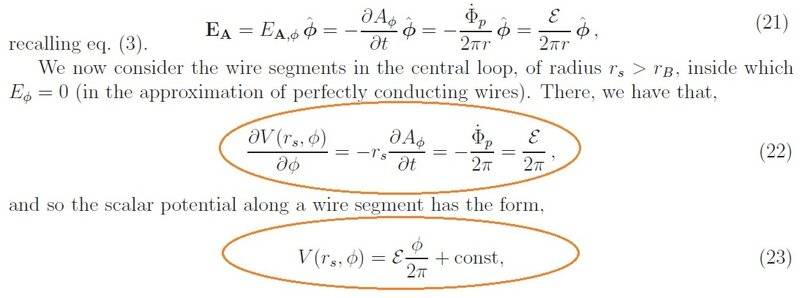Equation 22 describes the induced electric field strength, which is constant at every point throughout the ring, and Equation 23 describes the electric potential, whose value increases with angle. Then why it can draw the conclusion of equation 23 from equation 22. Since the electric field inside the perfectly conducting wire is zero, it must be because the electric field generated by the charges accumulated on the surface of the wire cancels the induced electric field inside the wire. Then the potential generated by the accumulated charge on the surface of the wire should also increase proportionally with the increase of the angle. This is consistent with what I've been saying. I believe it is difficult for each side to understand what the other is thinking without separately considering the electric field created by the accumulated charge on the surface of the wire.

Gold Member
2022 Award
There is no decomposition of the E-field involved. All you need is Faraday's Law as shown in #34.

Gold Member
2022 Award
@vanhees71
I'm not trying to describe the non-existent potential created by the induced electric field, but the scalar field created by the accumulated charge on this circuit.

As is the case in this paper http://kirkmcd.princeton.edu/examples/lewin.pdf , the same circuits are also described with potentials. Quoted below.

It's described by a scalar AND a vector potential, and that must be so, because the electric field is not a conservative field in this case, due to Faraday's Law!

alan123hk
Some references for the surface charge of current-carrying conductors.
Includes a demonstration of an electric field that actually exists in the surrounding space outside a current-carrying conductor.
https://physicsteacher.blog/tag/surface-charges-on-current-carrying-conductor/
https://zjui.intl.zju.edu.cn/course/ece329/Secure/LectureNotesforCalendar/optional/Jefimenko62.pdf

Can't we then try to describe and calculate the real electric field produced by a current-carrying conductor?

Last edited:
Homework Helper
2022 Award
Some references for the surface charge of current-carrying conductors.
Are these published in peer-reviewed format anywhere?

Gold Member
2022 Award
It's in any textbook on electromagnetism, and you don't need to rely on peer-review, if you calculate it yourself.

The most simple case is a very long coaxial cable. with a battery on one end and a resistor (or a short circuit) on the other. Then (in non-relativistic approximation) you can make the ansatz that ##\vec{j}=j \vec{e}_3## (where the cable is along ##\vec{e}_3##) with ##j=\text{const}## along the wires and ##0## outside.

Then you can solve the static Maxwell equations together with the appropriate boundary conditions and get the surface charges along the surfaces as well as the electric and magnetic fields everywhere.

alan123hk
simple question. In the following system, due to the induced electric field generated by the inductance, there must be only one electric field, which is a non-conservative field.
But how could there possibly be a faster and easier way to find an approximation of this non-conservative field without separately considering the electric field generated by the charges on the two plates of the capacitor?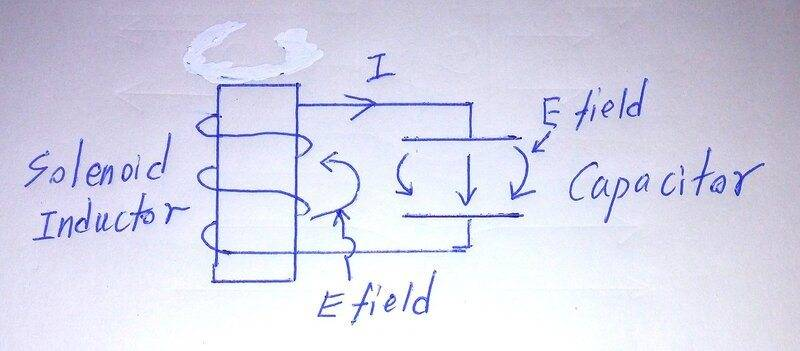Gold Member
2022 Award
You just use the usual quasistationary approximations of circuit theory and Faraday's Law then leads to
$$L \dot{i}=-CQ.$$
Using ##\dot{Q}=i##, you get
$$L \ddot{Q}=-C Q \; \Rightarrow \; \ddot{Q}=-\omega^2 Q, \quad \omega=1/\sqrt{LC}$$
The solution is
$$Q(t)=c_1 \cos(\omega t) + c_2 \sin(\omega t),$$
with the integration constants ##c_1## and ##c_2## given by the initial condition, i.e., ##Q(0)=Q_0## and ##i(0)=\dot{Q}(0)=i_0##.

alan123hk
This is just to find out the current, voltage and the amount of charge on the capacitor . I mean find out the electric field in all the surrounding space. The electric field in the entire space includes the induced electric field generated by the inductor and the electric field generated by the capacitor. They are stacked together.

I admit that there is only one non-conservative field, but on the other hand, how can there be a faster way to find this non-conservative field (even an approximation) directly without considering the electric fields generated by the inductor and capacitor separately?

Last edited:
Homework Helper
2022 Award
This is just to find out the current, voltage and the amount of charge on the capacitor . I mean find out the electric field in all the surrounding space. The electric field in the entire space includes the induced electric field generated by the inductor and the electric field generated by the capacitor. They are stacked together.

I admit that there is only one non-conservative field, but on the other hand, how can there be a faster way to find this non-conservative field (even an approximation) directly without considering the electric fields generated by the inductor and capacitor separately?
In order to find a useful approximate solution, one must rigorously define the purpose and range of the approximation, and never lose sight of the validity thereof. Much good physics has been done by making assumptions and rigorously deriving the results thereof.
Hans Bethe, for instance, was famous for making the correct ansatz in an otherwise intractible situation. But he never lost sight of the limitations and sometimes figuring out why such an ansatz works better than expected reveals hidden details.

•vanhees71
SDL
••cnh1995, alan123hk and vanhees71
alan123hk
Thank you for your replies, I think I need some time now to sort out and think about related issues.

•SDL
Homework Helper
2022 Award
•SDL and vanhees71
alan123hk
Maybe this reference would help:
https://web.mit.edu/6.013_book/www/chapter10/10.1.html See "Example 10.1.2. Electric Field of a One-Turn Solenoid".
Thanks for the link, Example 10.12 demonstrates how to use good mathematical techniques to derive analytical solutions to physics problems.

Below I try to find potential and potential difference using the simple concept I've been talking about. It is to regard the entire cylindrical structure as a circuit to calculate. Of course, this does not have the ability to find the electric field distribution in the entire space, since Laplace's equation and other more advanced mathematics have to be used.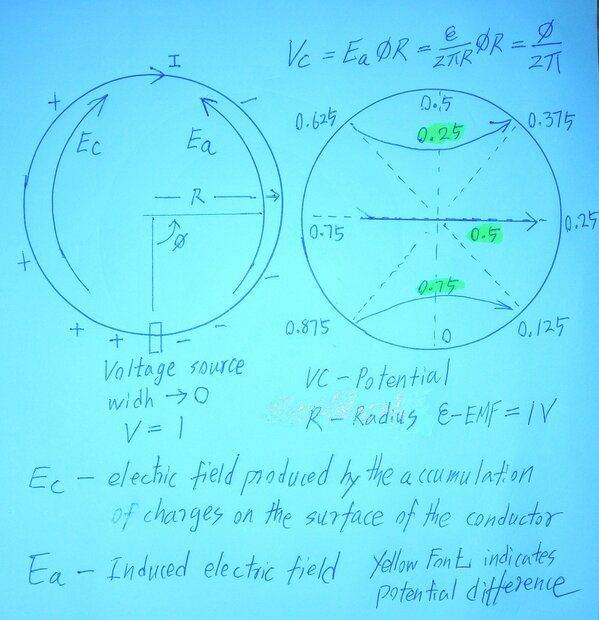Last edited:
Homework Helper
2022 Award
Of course, this does not have the ability to find the electric field distribution
Which is unique. QED.

alan123hk
I'm not trying to prove anything in this discussion thread, I'm just describing a concept and a simple calculation method. If people question or even thinks this is wrong, I'll try to explain it.

I should also mention about that the so-called build-up of charge on the surface of the wire creates an electric field that counteracts and cancels the induced electric field inside the wire. If there is a curl of electric field inside the wire, it cannot be eliminated. In this case, the curl of electric field creates large eddy currents inside the wire, resulting in energy losses (ohmic losses and radiation, etc.). In other words, it can only be said that this cancellation works in a fixed direction and very localized region of space.

Last edited:
Homework Helper
2022 Award
If people question or even thinks this is wrong, I'll try to explain it.
Because we may consider the method incorrect does not necessarilly mean we misunderstand your method.
If we do misunderstand please explain, but please seriously consider the other possibility.

•weirdoguy and vanhees71
alan123hk
The description of example 10.12 (https://web.mit.edu/6.013_book/www/chapter10/10.1.html) may be considered wrong, because the induced electric field is generally a time-varying field, so all other electric fields correspondingly generated in the system are time-varying, it cannot be accurately described by Laplace's equation. This is because Laplace's equation only applies to regions that do not contain charge, current, or time-dependent electromagnetic phenomena.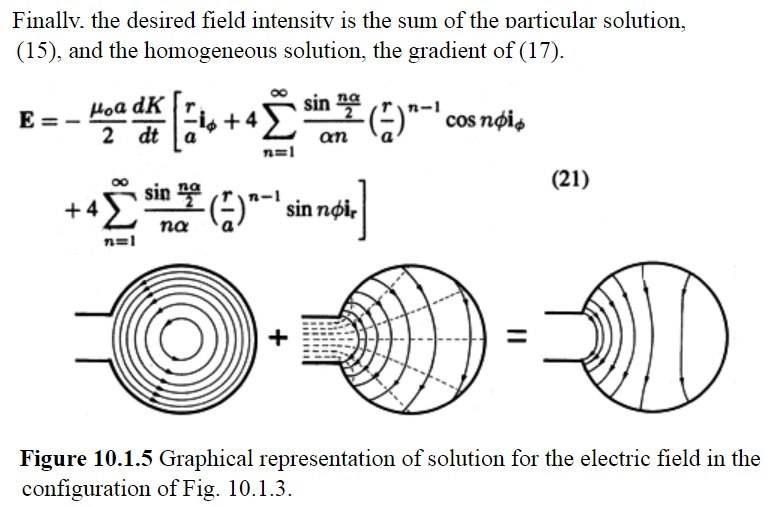However, this is probably a matter of opinion. Since the author uses Laplace's equation, it has been implied that the rate of change of the current should be a constant to get an accurate answer. Even if it is not constant, as long as the rate of change is low enough or the frequency is low enough, an approximation can be obtained. If the error in this approximation is considered acceptable, then it is a correct calculation.

Last edited:
Homework Helper
2022 Award
The title of the chapter might be a clue.

## PerfectConductors​

•vanhees71
alan123hk
Similarly, in the following equation, if the charge distribution and current are constant or change very slowly, all the electric fields generated by the charges can be approximately described by the conservative fields ##~-\nabla ~ \theta~##, and the term ##-\frac {\partial A} {\partial t}## only represents the induced electric field.
$$\\ E=-\nabla ~ \theta-\frac {\partial A} {\partial t}=E_c+E_a$$ If ## -\frac {\partial A} {\partial t} ## to represent the only non-conservative field ##E ~##that actually exists. Then it will become as follows. $$E= E_c+E ~~~~~\Rightarrow~~~~~E_c=0$$